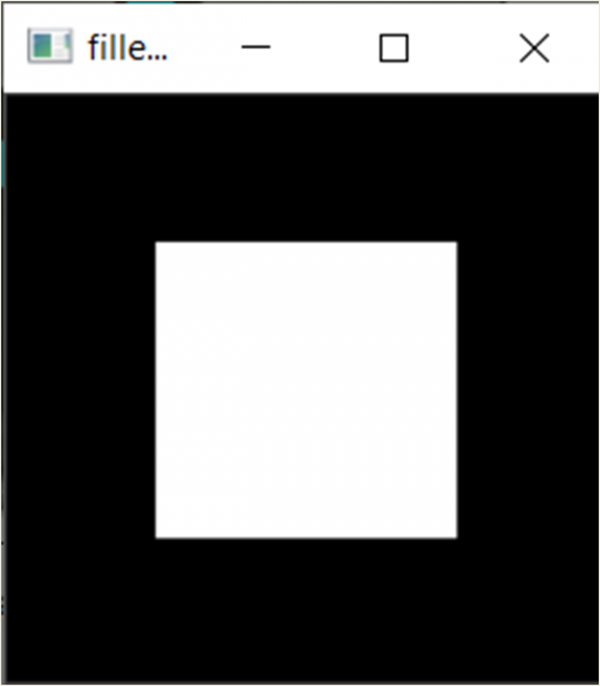# Draw a filled polygon using the OpenCV function fillPoly()

In this program, we will draw a filled polygon using the opencv function fillPoly(). The function takes in an image and the endpoints of the polygon.

## Algorithm

Step 1: Import cv2 and numpy.
Step 2: Define the endpoints.
Step 3: Define the image using zeros.
Step 4: Draw the polygon using the fillpoly() function.
Step 5: Display the output.

## Example Code

import cv2
import numpy as np
contours = np.array([[50,50], [50,150], [150,150], [150,50]])
image = np.zeros((200,200))
cv2.fillPoly(image, pts = [contours], color =(255,255,255))
cv2.imshow("filledPolygon", image)

## Output